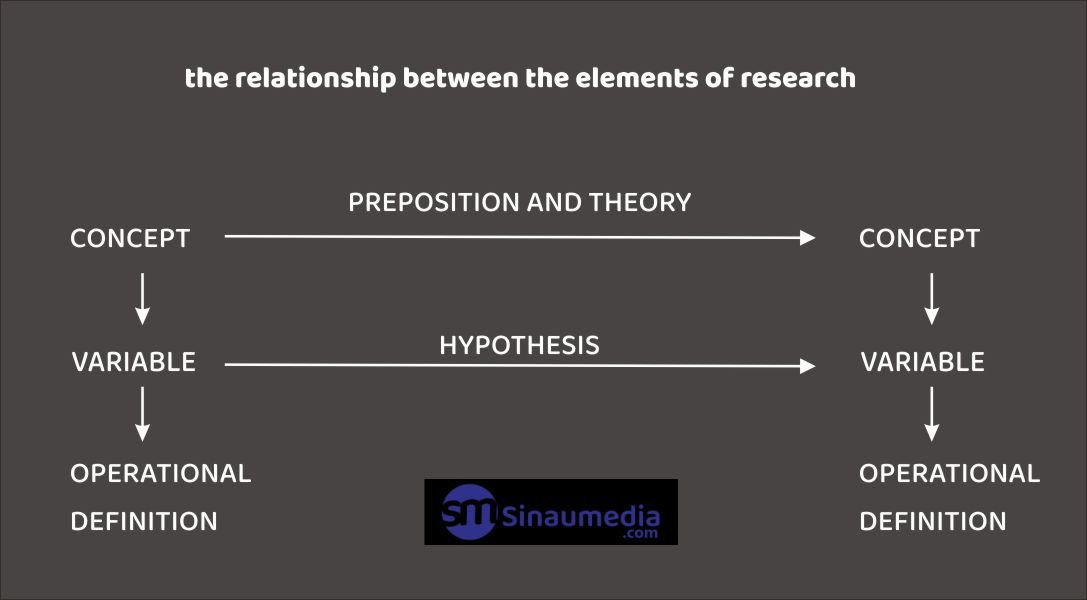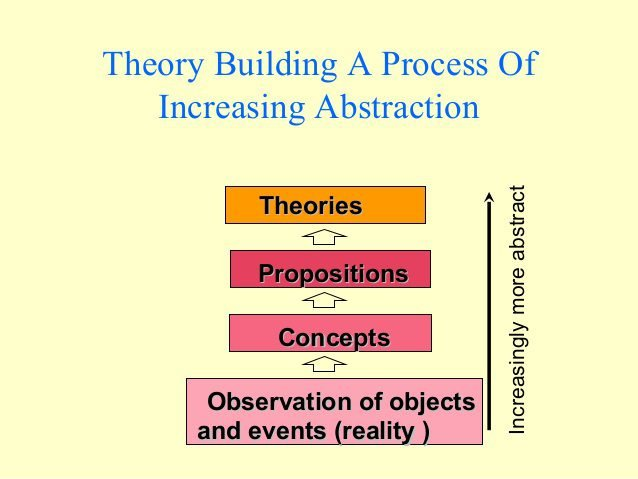# ELEMENTS OF SCIENTIFIC RESEARCH THAT MUST BE UNDERSTOODHello statisticians. Are you working on a script or want to take a script? Now this time sinaumedia will discuss the elements of scientific research. Keep on reading.

To be able to conduct research well, researchers need to have knowledge of various elements of research . The elements that form the basis of this scientific research are: concepts, prepositions, theories, variables, hypotheses and operational definitions .

Scientific research is a systematic process of proving the relationship. Therefore, the relationship between the elements of scientific research can also be presented systematically below.the relationship between the elements of research

The schematic above shows the theoretical process of the empirical process of a research; formulation of concepts, positions and theoretical arrangements in a scientific research. Formulation of operational definitions, data collection, formulation and testing of statistical hypotheses is an empirical process.

It should be remembered that the research process is an ongoing process and begins with the researcher’s deep interest in the relationship to be studied.

With the interest and ideas, the research process increases in theoretical understanding (which requires an understanding of concepts, theoretical propositions), hypothesis formulation (including an understanding of hypothetical variables, operational definitions and statistical hypotheses), and hypothesis testing.

## ELEMENTS OF SCIENTIFIC RESEARCH

Below, the statistical consultant’s efforts will describe the elements of this research one by one with examples of their actual use.

### 1. CONCEPT

The concept of research is the most important element of research and is the definition used by researchers to abstractly describe a social phenomenon or natural phenomenon.

For example, to describe the reproductive capacity of poultry known as the concept of fertility and hatchability. Another example to illustrate population movement is known as the concept of migration and mobility.

Some of the concepts commonly used in population research and social research include the value of children, contraceptive behavior, labor force, unemployment and so on.

### 2. PREPOSITION

Prepositions are statements about the nature of reality that can be tested for truth. A hypothesis is a proposition formulated for empirical testing. Theorems or laws are also prepositions that have a wider range or scope and have received a lot of empirical support.

### 3. THEORY

Theory is the main means of expressing the systematic relationship between social and natural phenomena to be studied. A theory is a logical sequence of one or more prepositions.

Is scientific information obtained to increase the abstraction of meanings and relationships in prepositions. Theory is scientific information obtained by increasing the abstraction of meanings and relationships in prepositions. More complex theories are usually logical combinations of several prepositions.### 4. VARIABLE

Another element commonly known as the element of research is the variable. Variable is a concept that has a variety of values.

So, the concept of the body is not a variable why and does not contain the meaning of the existence of varying values. Weight or height are variables because they have different values.

Sex is a variable because it can have a value that is male or female. Age, education, marital status, number of children, home ownership status, money supply are all variables.

Concepts that do not contain multiple value meanings can usually be turned into variables by focusing on certain aspects of the concept.

An example is eggs. The concept of eggs can be used as a variable, for example by assessing aspects of egg weight, egg length and width, egg color and so on.

### 5. HYPOTHESIS

As previously discussed, the research objective is to examine the systematic relationship between the variables. This relationship is usually presented in the form of a hypothesis which is an important research element.

A hypothesis is a tentative conclusion or preposition about the relationship between two or more variables. The hypothesis is always presented in the form of a statement that links explicitly or implicitly a variable with one or more other variables.

A good hypothesis must meet 2 criteria. First the hypothesis must describe the relationship between the variables. Both hypotheses should provide clues on how to test the relationship. This means that the variables listed must be measurable and the direction of the relationship between these variables must be clear.

Example of a hypothesis in animal husbandry research: the fertility rate of native chicken eggs is influenced by the quality of feed and the age of the mother.

### 6. OPERATIONAL DEFINITION

One element of research that really helps communication between researchers is the operational definition. The operational definition is an indication of how a variable is measured. By reading the operational definition of a study, a researcher will know the measurement of a variable, can know the good or bad of the measurement.

In the operational definition section, it is usually explained how the research method is carried out. such as the number of samples, how to measure variables, the research process, experimental design, how to analyze statistical data, and so on.